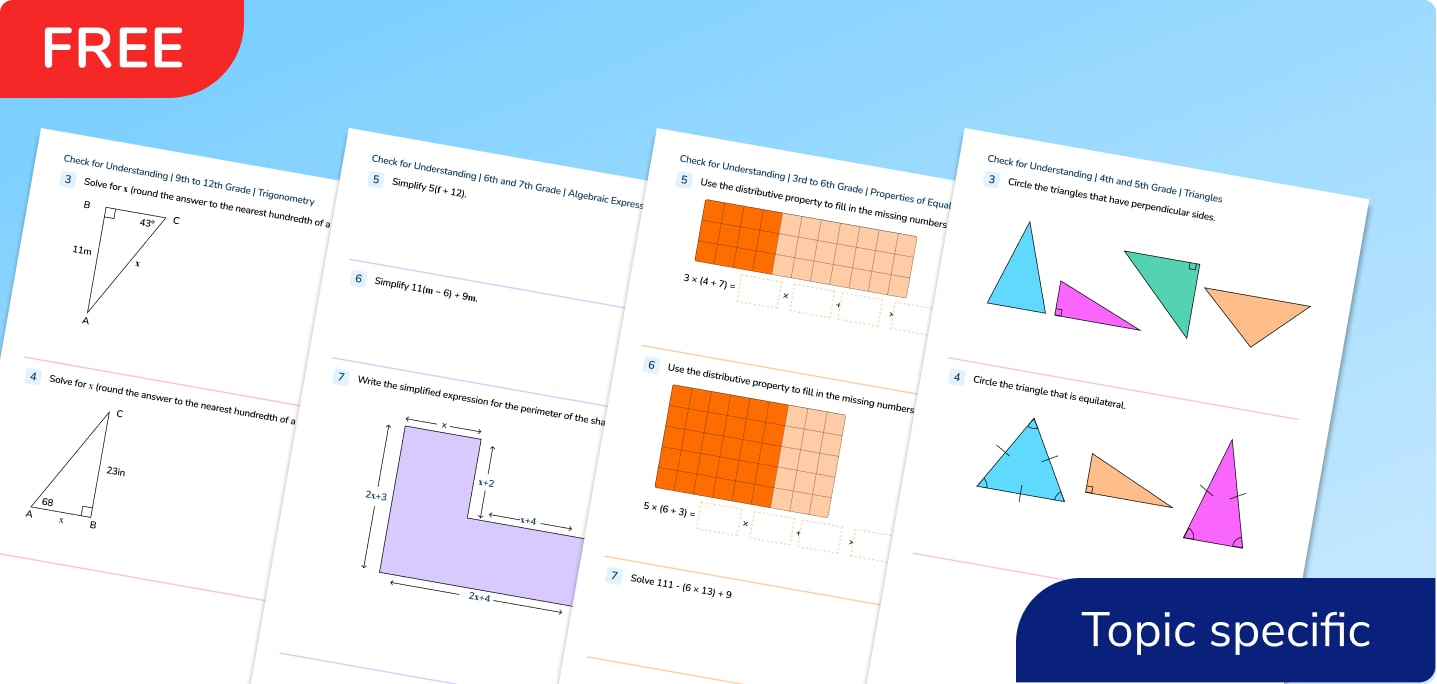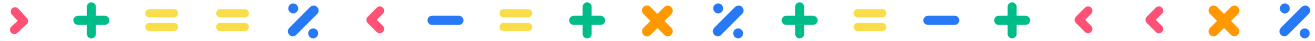Math Resources

# Quizzes

Free topic specific math quizzes to help check your students’ understanding of topic areas.What can you expect from the quizzes?

Identify your students' areas of strength and where support is needed with topics.

Written by math experts.

Filter by:

Kintergarten

Mixed

Counting and Cardinality

Operations and Algebraic Thinking

Number and Operations in Base Ten

Number and Operations - Fractions

The Number System

Measurement and Data

Geometry

Ratios and Proportional Relationships

Expressions and Equations

Statistics and Probability

Functions

The Real Number System

Quantities

The Complex Number System

Vector and Matrix Quantities

Seeing Structure in Expressions

Arithmetic with Polynomials and Rational Expressions

Creating Equations

Reasoning with Equations and Inequalities

Interpreting Functions

Building Functions

Trigonometric Functions

Congruence

Similarity, Right Triangles and Trigonometry

Circles

Expressing Geometric Properties with Equations

Geometric Measurement and Dimension

Modeling with Geometry

Interpreting Categorical and Quantitative Data

Making Inferences and Justifying Conclusions

Conditional Probability and the Rules of Probability

Using Probability to Make Decisions

Clear filter

## Can't find what you're looking for?## Personalized one-on-one math instruction made easy

At Third Space Learning, we’re on a mission to help teachers like you shape your students into confident, able mathematicians.

Request a demo